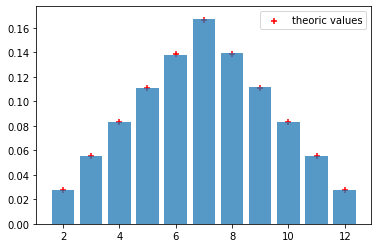# Statistics Simulation

Last updated: September 3rd, 2020# Statistics simulation¶

#### EXERCISES¶

1- Make a program that simulates flipping a coin N times. We want to know the number of heads we will observe if toss the coin 10 times

In :
import numpy as np
import pandas as pd
import random
import matplotlib.pyplot as plt

In :
# 1-Make a program that simulates flipping a coin N times. Print out tail or head for each flip and let the program count and print the number of heads.
np.random.seed(42)
n = 10
p = 0.5
np.random.binomial(n, p)

Out:
4

2- Obtain the distribution of the random variable 'sum' of the result of rolling two dice.

In :
# Obtain the distribution of the random variable sum of the result of rolling two dice.

sample_1 = np.random.randint(1,7, size = 1000000)
sample_2 = np.random.randint(1,7, size = 1000000)

summ = sample_1 + sample_2

result = np.arange(2,13)
theoric_values = np.array([1,2,3,4,5,6,5,4,3,2,1])/36

plt.hist(summ, bins = np.arange(1.5,13.5,1), density=True, rwidth = 0.8, alpha = 0.75)
plt.scatter(result, theoric_values, marker = '+', c = 'red', label = 'theoric values')
plt.legend()
plt.show()3- The computer determines a secret number, and the player shall guess the number. For each guess, the computer tells if the number is too high or too low. We let the computer draw a random integer in an interval known to the player, let us say [1,100]. In a while loop the program prompts the player for a guess, reads the guess, and checks if the guess is higher or lower than the drawn number. An appropriate message is written to the screen.

In [ ]:
import random
number = random.randint(1, 100)
attempts = 0  # count no of attempts to guess the number
guess = 0
while guess != number:
guess = eval(input('Guess a number: '))
attempts += 1
if guess == number:
print ('Correct! You used', attempts, 'attempts!')
break
elif guess < number:
print ('Go higher!')
else:
print ('Go lower!')


4- Generate a random normal distribution of size 2x3

In :
x = np.random.normal(size=(2, 3))
print(x)

[[ 0.49671415 -0.1382643   0.64768854]
[ 1.52302986 -0.23415337 -0.23413696]]


5- Generate a random normal distribution of size 2x3 with mean at 1 and standard deviation of 2

In :
x = np.random.normal(loc=1, scale=2, size=(2, 3))
print(x)

[[4.15842563 2.53486946 0.06105123]
[2.08512009 0.07316461 0.06854049]]


6- Generate a random 1x10 distribution for occurence 2

In :
x = np.random.poisson(lam=2, size=10)

print(x)

[2 2 1 2 2 1 0 4 2 1]